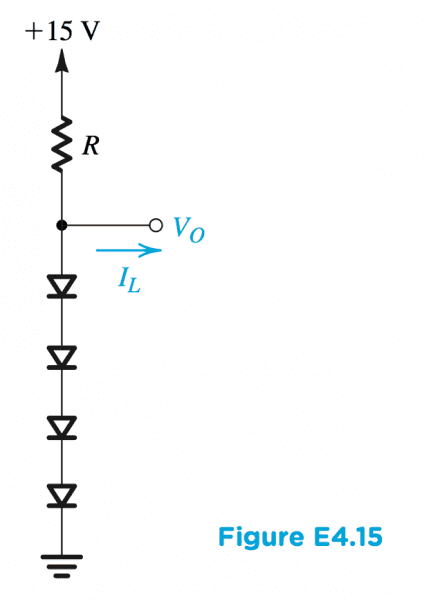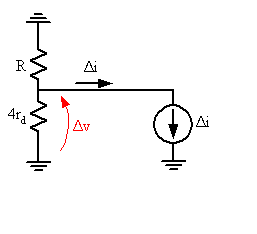# Design the diode circuit

• Engineering
• STEMucator
This equation can be rearranged to solve for ##r_d##:$$r_d = 4 V_T/I_{r_d}$$The answer is ##2.4k##.In summary, in the small signal model, the load current is the signal and the voltage at the node above the diodes is 3 V. The current across the diodes is given by the equation i = (V o /r d )/(4 V_T/i_d).f

Homework Helper

## Homework Statement

Design the circuit in the following figure so that ##V_o = 3V## when ##I_L = 0## and ##V_o## changes by ##20mV## per ##1mA## of load current.

(a) Use the small-signal model of the diode to find the value of ##R##.
(b) Specify the value of ##I_S## (saturation current) of each of the diodes.## The Attempt at a Solution

I don't understand how part (a) is to be accomplished. Assuming a diode current ##I_D## flows from top to bottom, the current can be found from:

$$I_D = \frac{15V - 3V}{R}$$

The small signal resistance is given by:

$$r_d = \frac{V_T}{I_D}$$

The answer is listed as ##2.4k##, but I am unsure how to proceed.

Last edited:
Okay, so you have an expression relating the operating point current ##I_D## and the resistance R. You should also be able to determine what the diode voltage ##V_D## has to be in order for the output to be at 3 V under no load. (It looks pretty high for a silicon diode that must be passing a fairly modest current, so we can anticipate that the diode saturation current is going to be pretty darn small).

Next I think you'll want to turn to the small signal model. Maybe write a nodal equation at the Vout node. The voltage there will be the Δv that results from a load current Δi. See if you can't arrange the equation to equate Δv/Δi to something involving the unknown resistances ##r_d## and R since you're given some information about what you want Δv/Δi to be. Your expression for ##r_d## in your post will let you bridge these results back to the large signal stuff by getting rid of ##r_d## using ##V_T/I_D##.

See where that takes you.

If I wanted ##V_o = 3V##, would I not say ##V_D = 0.75V## for each diode in series?

The small signal model consists of a source ##v_s## in series with the resistor ##R## and the small signal resistance ##r_d## (the series combination of the diodes). I don't think I can write a node equation for this series circuit, did you mean a voltage divider?

If I wanted ##V_o = 3V##, would I not say ##V_D = 0.75V## for each diode in series?
Yup.
The small signal model consists of a source ##v_s## in series with the resistor ##R## and the small signal resistance ##r_d## (the series combination of the diodes). I don't think I can write a node equation for this series circuit, did you mean a voltage divider?
The only source in the small signal model will be the load current. The 15 V DC source is suppressed, and it never had an AC signal component.

Yup.

The only source in the small signal model will be the load current. The 15 V DC source is suppressed, and it never had an AC signal component.

Ah, short circuit the voltage and open circuit the current, sorry it's pretty early. So the DC analysis has already been done.

The small signal model has a resistor ##R## in series with ##r_d## and a common ground.

Since ##I_L = 0##, the voltage at the node above ##r_d## is ##V_o = 3V##. Does that mean the current across ##r_d## is ##i_d = \frac{V_o}{r_d}##?

I'm still a bit lost, i'll try after getting some sleep.

In the small signal model the Vo = 3 V disappears. That's a DC operating point condition and doesn't enter into the small signal model. The load current is of interest in the small signal model though, it is the "signal" in the small signal model. So what you're left with is the resistance R, the diode small signal resistances rd, and a load current as the signal. I know it seems strange to have the load as a signal, but it's what is varying according to the problem. Here's what we're looking at:In the small signal model the Vo = 3 V disappears. That's a DC operating point condition and doesn't enter into the small signal model. The load current is of interest in the small signal model though, it is the "signal" in the small signal model. So what you're left with is the resistance R, the diode small signal resistances rd, and a load current as the signal. I know it seems strange to have the load as a signal, but it's what is varying according to the problem. Here's what we're looking at:

View attachment 78753

I thought about replacing the diodes with ##4 r_d## in the model before I slept, but I didn't know if it had any merit. So it turns out the load current IS the signal in the small signal model.

So writing a nodal equation at the only node in the circuit:

$$I_R - I_{r_d} - \Delta i = 0 \Rightarrow \frac{0 - \Delta v}{R} - \frac{\Delta v - 0}{4 r_d} = \Delta i \Rightarrow - \frac{\Delta v}{R} - \frac{\Delta v}{4 r_d} = \Delta i$$

So we can conlcude:

$$\Delta v \left[- \frac{1}{R} - \frac{1}{4 r_d} \right] = \Delta i \Rightarrow \frac{\Delta v}{\Delta i} = - \frac{1}{\left[ \frac{1}{R} + \frac{1}{4 r_d} \right]} \Rightarrow \frac{\Delta v}{\Delta i} = - \frac{4 R r_d}{R + 4r_d}$$

Substituting in for ##r_d = \frac{V_T}{I_D} = \frac{V_T}{\frac{12 V}{R}} = \frac{(25 \times 10^{-3} V)R}{12 V} = 0.002083 R##:

$$\frac{\Delta v}{\Delta i} = - \frac{4 R (0.002083 R)}{R + 4(0.002083 R)} = - 0.00826315 R$$

So we know ##\frac{\Delta v}{\Delta i} = \frac{20 mV}{1 mA} = 20 \Omega##, hence:

$$R = \frac{20 \Omega}{0.00826315} = 2420.4 \Omega = 2.42 k \Omega$$

The only thing bothering me at this point is why there's a negative sign, which I had to ignore (since ##|R| \geq 0##). Is this relevant?

Sleeeep <3

EDIT: I forgot to mention the saturation current:

$$I_S = \frac{I_D}{e^{\frac{V_D}{V_T}}} = \frac{\frac{12 V}{2.42 \times 10^3 \Omega}}{e^{\frac{0.75 V}{25 \times 10^{-3} V}}} = 4.64 \times 10^{-16} A$$

Last edited:
Well done! Just a little niggle:

The only thing bothering me at this point is why there's a negative sign, which I had to ignore (since |R|≥0|R| \geq 0). Is this relevant?

Do a little thinking about Δv/Δvi. The problem statement says that Vout changes by 20 mV per 1 mA of load current. It doesn't say it which direction it changes. Reflect on what should happen to Vout as the load draws more current.

Well done! Just a little niggle:

Do a little thinking about Δv/Δvi. The problem statement says that Vout changes by 20 mV per 1 mA of load current. It doesn't say it which direction it changes. Reflect on what should happen to Vout as the load draws more current.

Ah I see, so as the load current ##I_L## increases by ##1mA## at a time, the output voltage ##V_o## is going to decrease. Hence why the negative sign was there.

Ah I see, so as the load current ILI_L increases by 1mA1mA at a time, the output voltage VoV_o is going to decrease. Hence why the negative sign was there.
Yes indeed!